# Approximating feature advantage of the Symmetric Difference Quotient over the Divided Difference

In numerical differentiation, symmetric Newton’s quotient (a.k.a. symmetric difference quotient) is known to more effectively approximate the first derivative of given function, in comparison with the (first-order) divided difference. This statement can be summarized as the following proposition.

Proposition 1.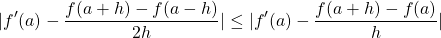for sufficiently small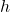and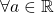.

This sounds intuitively correct, since taking average of left and right divided differences at the point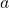seem to smoothen the drastic slope change that can occasionally occur only one side. This is shown by somewhat technical manner.

Proof. Note that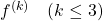is bounded aroundsinceis assumed to be of class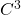as a function on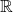. By the Taylor series expression of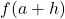and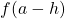at, we get: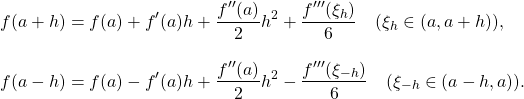Subtracting the second row from the first, and divided by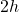yealds: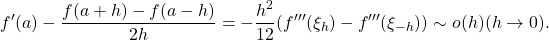Similarly we have the lower order infinitesimal equation: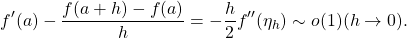By takingand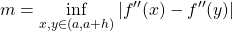, we can evaluate the equation only by the order of infinitesimal with regard toand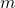being constant whenis sufficiently small, which finish the proof ■